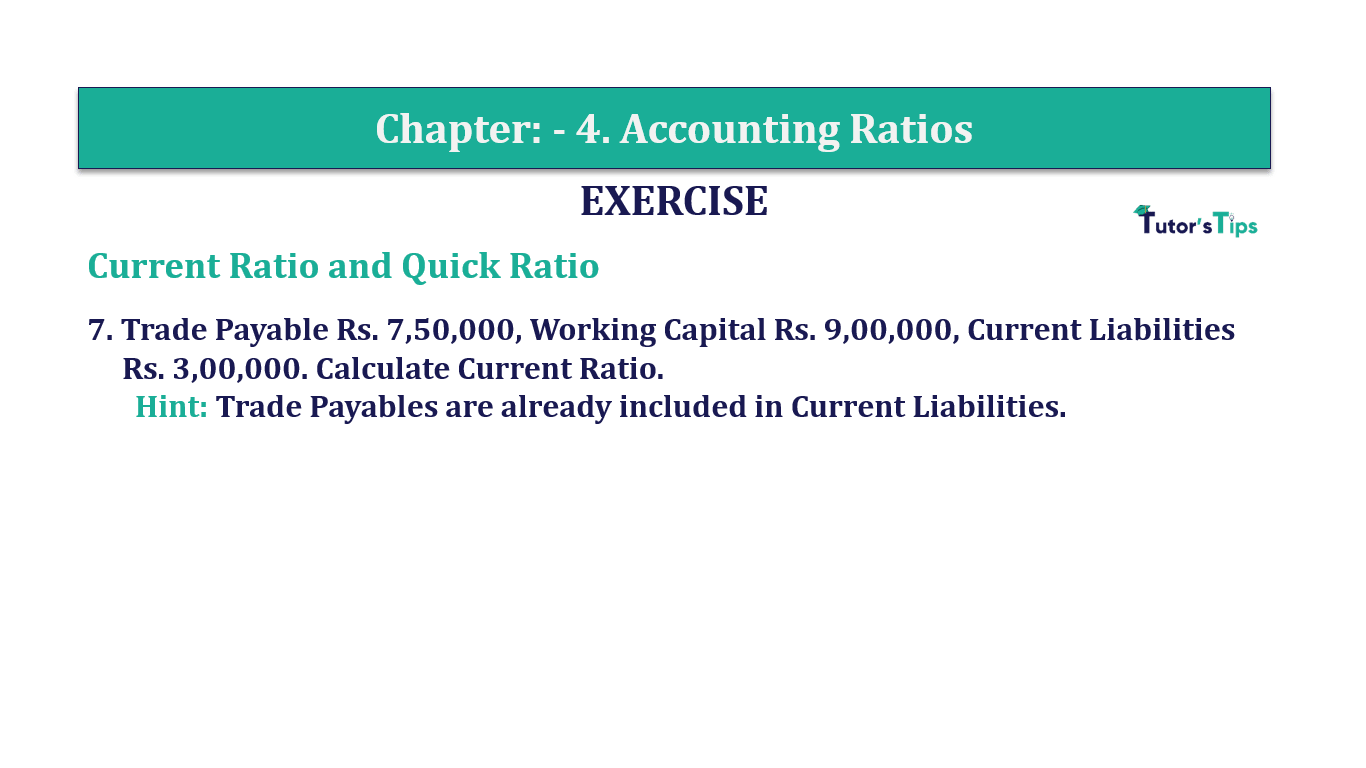# Question 7 Chapter 4 of +2-B – T.S. Grewal 12 ClassQuestion No. 7 - Chapter No.4 - T.S. Grewal +2 Book Part B

Question 7 Chapter 4 of +2-B

Current Ratio and Quick Ratio

7. Trade Payable Rs. 7,50,000, Working Capital Rs. 9,00,000, Current Liabilities
Rs. 3,00,000. Calculate Current Ratio.

### The solution of Question 7 Chapter 4 of +2-B: –

 Working Capital= Rs. 9,00,000
 Current Liabilities =Rs. 3,00,000
 Working Capital =Current Assets – Current Liabilities
 Current Assets= Rs. 9,00,000 + 3,00,000
 Current Assets =Rs. 12,00,000

 Current Ratio= Current Assets Current Liabilities
 Current Ratio= 12,00,000 3,00,000

Current Ratio=4 : 1

Balance Sheet: Meaning, Format & Examples

Comment if you have any question.

Also, Check out the solved question of previous Chapters: –